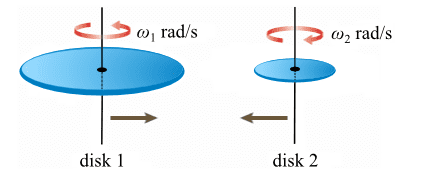# When does "no slipping" happens

## Homework Statement

Hey everyone

I'm studying for a test and I can't get this concept right in my headIf I bring both disks closer to each other, what exactly makes it so they're not slipping?

The question is this

Two disks are spinning freely about axes that run through their respective centers (see figure below). The larger disk (R1 = 1.42 m) has a moment of inertia of 1140 kg · m2 and an angular speed of 5.4 rad/s. The smaller disk (R2 = 0.60 m) has a moment of inertia of 920 kg · m2 and an angular speed of 8.0 rad/s. The smaller disk is rotating in a direction that is opposite to the larger disk. The edges of the two disks are brought into contact with each other while keeping their axes parallel. They initially slip against each other until the friction between the two disks eventually stops the slipping. How much energy is lost to friction? (Assume that the disks continue to spin after the disks stop slipping.)

## The Attempt at a Solution

I know momentum is conserved.

Initial momentum = I1 ω1 + I2 ω2

Kinetic energy is NOT conserved, so Energy Lost = Initial K - Final K

I would use K = 1/2 (I ω)

I know the angular speed also not the same at the end because they have difference radius.

How do I figure out when they're not slipping? What exactly does it mean when it says no slipping?

My best guess was that their tangential velocity is the same, because they're touching.

Initial momentum = Final momentum = 13516

13516 = I ω1f + I ω2f

R1 ω1f = R2 ω2f

ω1f = R2 ω2f / R1

13516 = I (R2 ω2f / R1) + I ω2f

ω2f = 9.66

ω1f = 4.05

Energy Lost = Initial K - Final K

Energy Lost = 0.5(1140 * 5.42 + 920 * 82) - 0.5(1140 * 4.052 + 920 * 9.662) = - 6213.4 J
I end up with a negative energy lost, which seems very wrong.

Thank you.

mfb
Mentor
My best guess was that their tangential velocity is the same, because they're touching.
Right.

Your disks are rotating in the opposite direction. This does not matter for the energy, but it is important for the momentum.

haruspex
Homework Helper
Gold Member
2020 Award
I know momentum is conserved.
I assume you mean angular momentum.
Bear in mind that each disk is on an axle, and presumably there are forces holding these axles steady.
Angular momentum is only meaningful in relation to a specified reference point. When there are unknown forces from outside the system, you can often make those irrelevant by choosing a reference point about which those forces have no moment. But in this case, no matter which axle you take as your reference, the force from the other axle will have a moment.
I suggest that instead you consider the free body diagram of each disk, putting in an unknown for the frictional force where they meet.

mfb
Mentor
Angular momentum is only meaningful in relation to a specified reference point.
As there is no net motion of any object, angular momentum is the same for all reference points here.

haruspex
Homework Helper
Gold Member
2020 Award
As there is no net motion of any object, angular momentum is the same for all reference points here.
That's true, and I should have mentioned that, but the important point is that you cannot use conservation of angular momentum here.

mfb
Mentor
Hmm, true, if two identical disks would both rotate in the same direction with the same angular velocity they would come to a halt, getting rid of all angular momentum via their supports. So we have to see how friction changes the angular velocities to find a new conserved quantity.